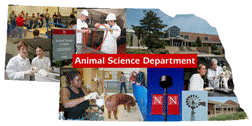## Animal Science Department## Faculty Papers and Publications in Animal Science

#### Date of this Version

1-14-2003

Published in J. Anim. Sci. 2003. 81:61–67.

#### Abstract

Fifteen models were compared for the birth weight of 33,994 lambs recorded at the U.S. Sheep Experimental Station (1950 to 1998). The initial intent was to estimate fractions of variance due to cytoplasmic line (c2; n = 892) and sire by cytoplasmic line interaction (sc2; n = 17,557). The basic model included direct genetic (fractional variance, a2; n = 35,684), maternal genetic (m2, with correlation r-am), and maternal permanent environmental (p2; n = 8,418) effects. The model with sc2 was significantly better than the basic model with and without c2. When other random effects were added, sc2 became zero. Significant effects were associated with random dam × year (dy2; n = 24,801), sire × dam (sd2; n = 23,924), and dam × number born (dn2; n = 12,944) interaction effects. Estimates with all effects in the model were a2, 0.24; m2, 0.19; r-am, 0.11; p2, 0.05; c2, 0.00; dn2, 0.04; dy2, 0.06; sd2, 0.05; sc2, 0.00. Estimates for a2, m2, and r-am were the same for all models. Estimate of p2 changed when other effects were added to the model. Largest estimates for nongenetic effects were: p2, 0.08; c2, 0.00; dy2, 0.13; sd2, 0.11; and sc2, 0.04. Regardless of whether Westell groups (n = 91) were in the model, estimates were similar. For weaning weight (120-d, n = 32,715), estimates of variances of effects added to the basic model were all near zero (a2, 0.18; m2, 0.12; r-am, −0.01; p2, 0.06). For number born (NB, n = 37,020) and fleece weight (FW, n = 36,197), animal permanent environmental effects were added to the model (ap2; n = 9,871 and 9,760) and r-am was dropped. For these traits, effects not in the basic model had small variances. Nonzero estimates with full model were a2, 0.10; ap2, 0.01; dy2, 0.02; and sc2, 0.01 for NB, and a2, 0.54; m2, 0.02; ap2, 0.02; dy2, 0.04; and sc2, 0.02 for FW. Cytoplasmic effects were not important. The addition of unusual random effects to the model did not change estimates for the basic parameters. Although some of these effects were significant, especially for BW, the effects on genetic evaluations are likely to be small.

COinS